Example  LInEQ.1 : Suppose $5x−10≤20$. Solve the inequality $x$.
Solution: Since $A> 0$, the inequality can be solved so that $x \le \frac {C- B} A$  where $A=5, B = -10$, and $C = 20$. So the solution is $x \le \frac {20 - (-10)} 5 = \frac {30} 5 = 6.$

Comment: We can consider the expression on the left hand side of the inequality as a function of $x$ giving $f(x) = 5x - 10$ . Now the problem can be restated: to find a $x$ where $f(x) \le 20$.
This problem and its solution can be visualized both on the graph and  the mapping diagram using the focus point.
 For the graph of $f$: Find $y\le20$ on the Y axis , then find the point on the graph of $f$ with second coordinate $20$, determine it's first coordinate, $6$, and that is the desired value for the solution that $x\le 6$. For the mapping diagram of $f$: Find $y=20$ on the target axis , then find $x$ on the source axis with the function arrow pointing to $20$ and that is the desired value for the solution that $x\le 6$.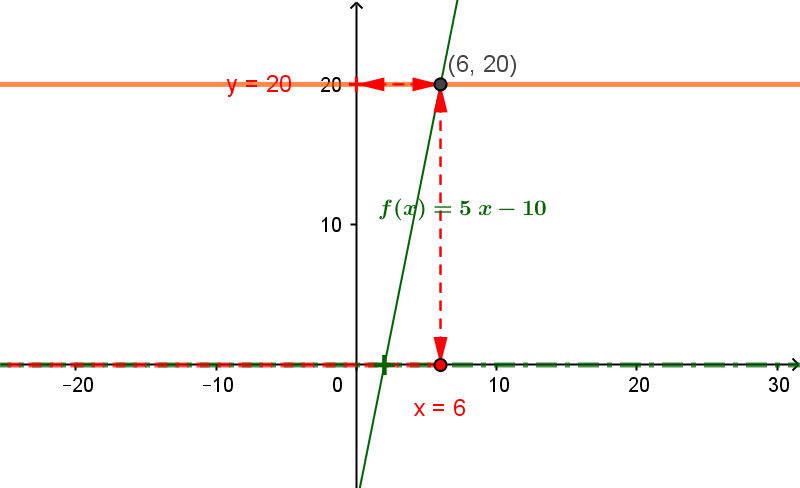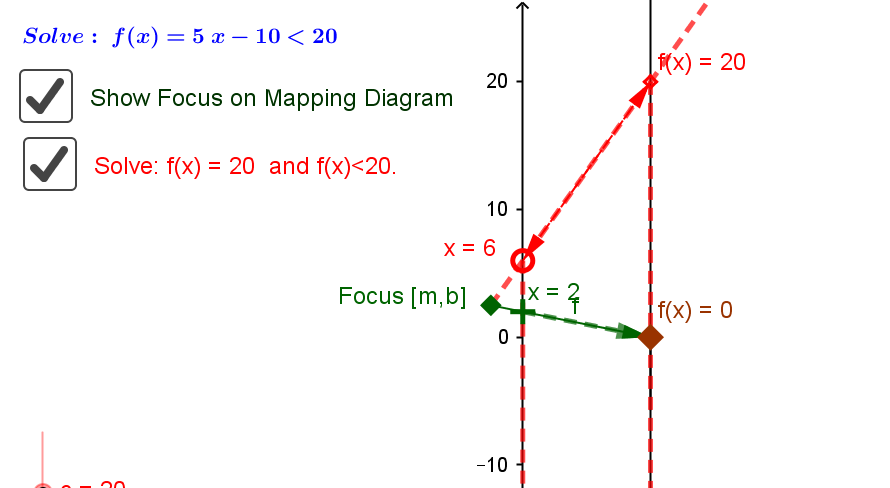To do this, look for the point where the line $y=20$ intersects the line that is the graph of $f$, namely $(6,20)$ giving the solution for $f(x) \le 20$ , that is $x\le 6$ or $x\in (-\infty,6]$. To do this,  find the focus point of $f$, $F= [5, -10]$ on the mapping diagram. Draw the line through F and the point $y=20$, to find the point of intersection of this line with the X axis, $x=6$, which is the desired value for $x$, giving the solution for $f(x) \le 20$ , that is $x\le 6$ or $x\in (-\infty,6]$.

Connecting the mapping diagram solution to the algebra:
Recognize that $f$ is a composition, $f(x)= s(m(x))$ where $m(x)=5x$ and $s(x)=x-10$.
The solution can be visualized more clearly connected to the algebra for solving the inequality with the mapping diagram for the function $f$ as a composition.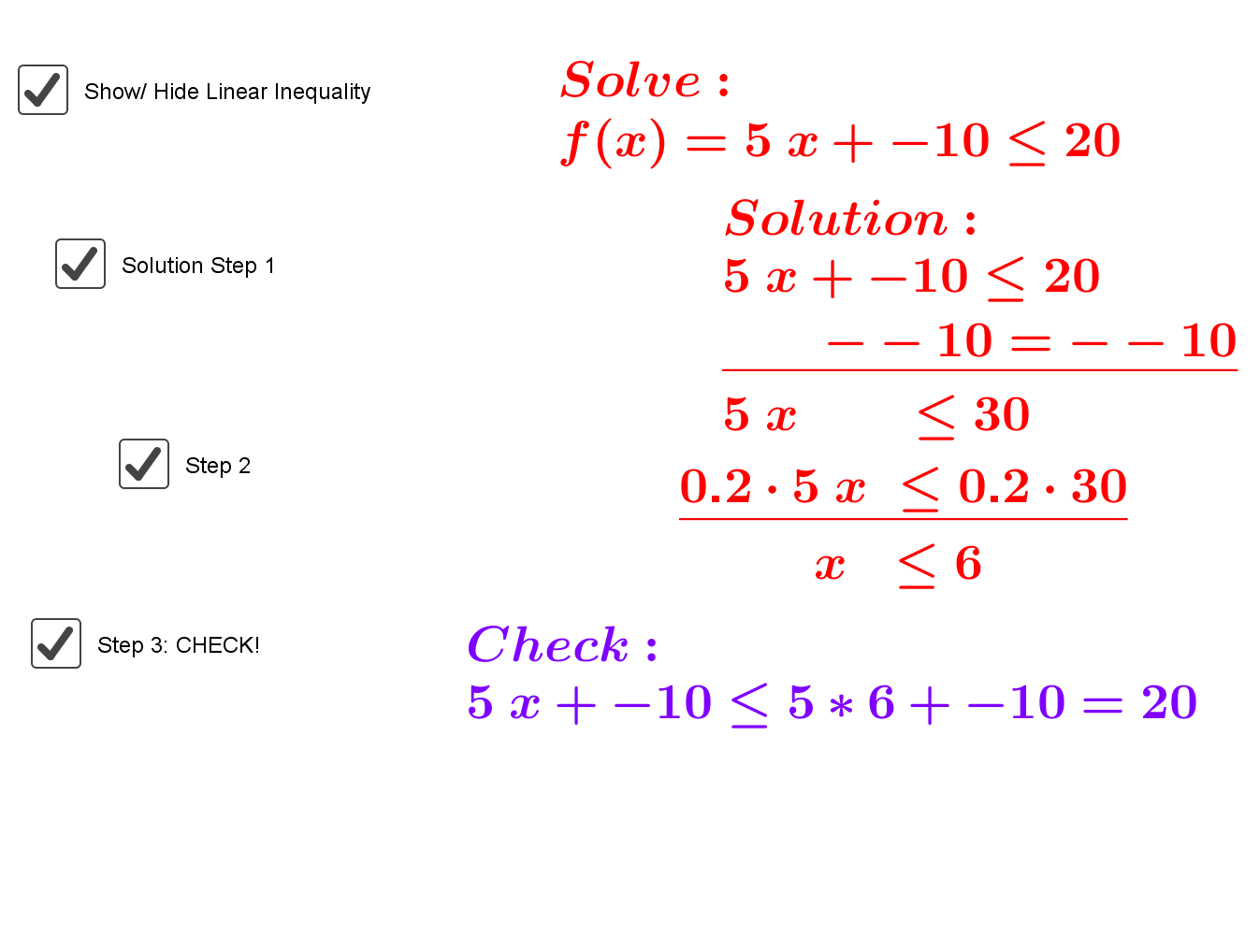On the mapping diagram, from the point $y=20$ on the target line, find the point on the middle axis, $u$, where $s(u)=20$. Visually this gives an arrow from the target axis to the middle axis that undoes the function $s$, which visualizes the inverse function for $s$, namely $u=s^{-1}(y)=y+10=20+10=30$.
Now we have that $u\le 30$ if and only if $s(u)\le 20$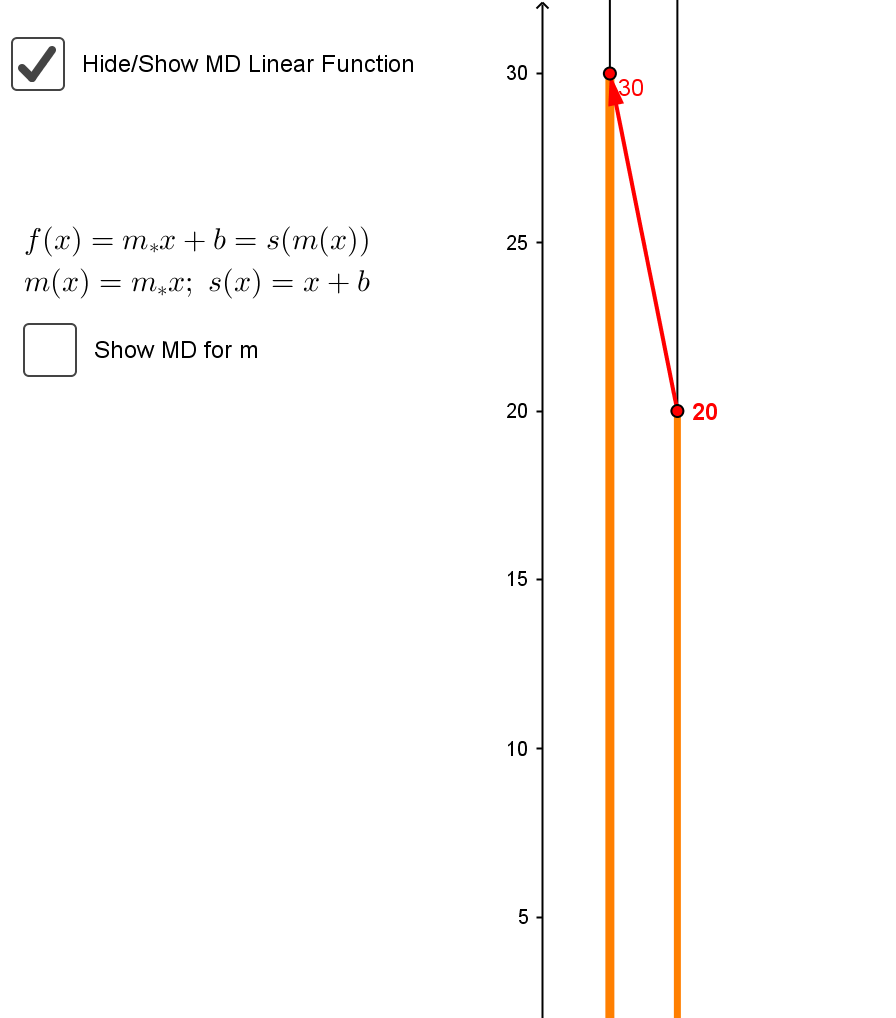To complete the solution find the point on the source axis, $x$ , where $m(x) = 30$.
Visually this gives an arrow from the middle axis to the source axis that undoes the function $m$, which visualizes the inverse function for $m$, namely $x=m^{-1}(u)=\frac15 u= \frac15 30=6$.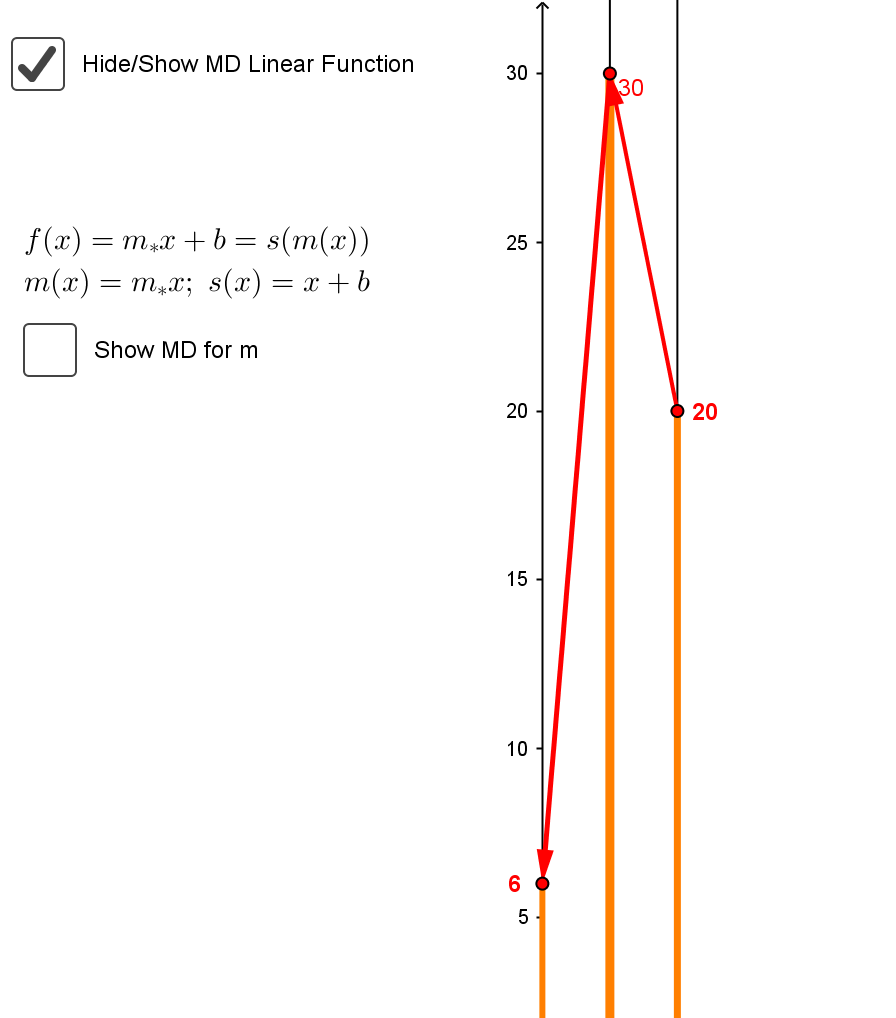Since the function $m(x)=5x$ is increasing, if $x\le 6, m(x) \le 30$. Thus we have that $x\le6$ if and only if $m(x)\le 30$ if and only if $f(x) = s(m(x))\le 20$
giving the solution for $f(x) \le 20$ , that is $x\le 6$ or $x\in (-\infty,6]$.